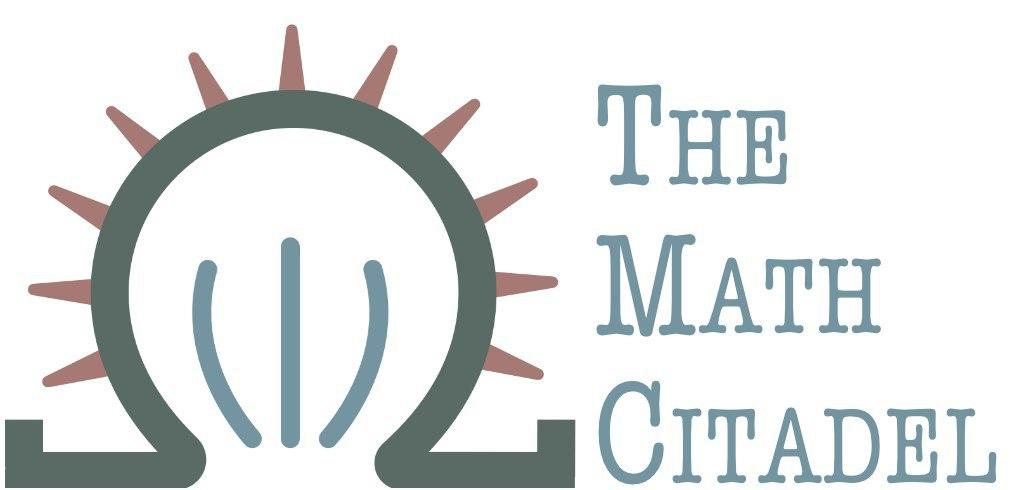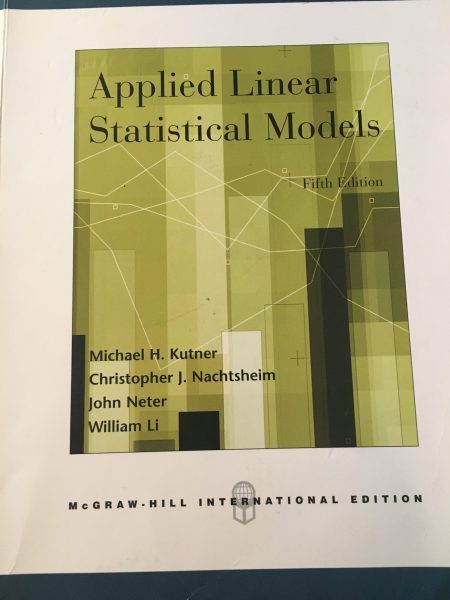## Book Review: Applied Linear Statistical Models, 5th ed.

### Author: M. Kutner, C. Nahtsheim, J. Neter, and W. Li

#### Reviewed by: R. Traylor## ISBN

• 0-07-112221-4

• ## Classification: Statistics and Data Science

• Hathlor code: M-STS.00110100

• ## Prerequisites

• solid grasp of and comfort with conventional algebra, some basic statistical concepts, calculus is a plus but not necessary

• ## Ranking

All ranking is done on a scale of 1-5, with 5 as the best.
Attribute Rank
Difficulty 2
Proof Quality NA
Self-Study 4
Good for Teaching? 5
Quality of Exercises 5

## Topics Covered

• Simple Linear Regression
• linear regression with one predictor
• inference in regression and correlation analysis
• diagnostics and remedial measures
• simultaneous inferences
• Multiple Linear Regression
• general linear model in matrix terms
• ANOVA results
• extra sums of squares and their use in regression tests
• dealing with qualitative and quantitative predictors
• model selection and validation, diagnostics, and remedial measures
• intro to time series data: autocorrelation
• Nonlinear Regression
• intro to nonlinear regression and neural networks
• logistic and poisson regression
• Design and Analysis of Single Factor Studies
• single-factor studies
• analysis of factor level means
• ANOVA diagnostics
• Multi-Factor Studies
• Two-Factor studies
• randomized complete block designs
• ANCOVA
• Multi-factor studies
• Random and Mixed Effects Models
• Specialized Study Designs
• nested designs
• released measure designs
• balanced incomplete block designs
• Factorial and fractional factorial designs
• response surface methodology